Pressure vessels

# Spherical pressure vessels:

Consider the stresses on one half of the thin spherical pressure vessel of inner radius r and wall thickness t.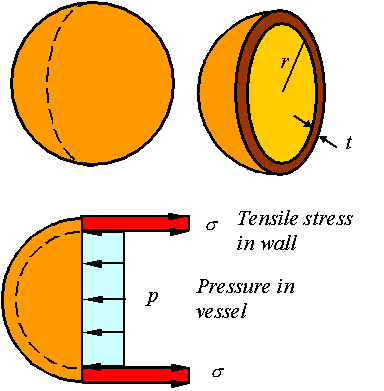Static equilibrium requires that the load generated from the tensile stress in the wall be equal to the load applied by the pressure. Since the vessel is thin, the load due to the tensile stress in the wall is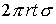. The load due to the pressure in the vessel is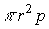. Balancing these gives the expression for the stress in a spherical vessel as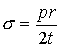Due to symmetry in the spherical pressure vessel, the stress in all directions tangent to the surface of the vessel is the same. Depending on weather one takes a stress element from the inside or outside surface of the vessel, one will get one of the two following states of stress.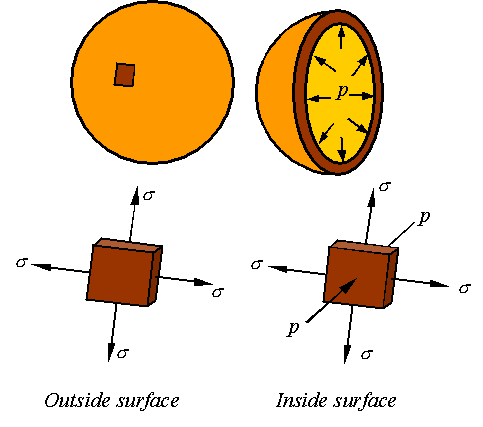Maximum stress on the outside surface:

The in and out-of-plane Mohr’s circles for a stress element taken from the outside surface of the pressure vessel will look as follows.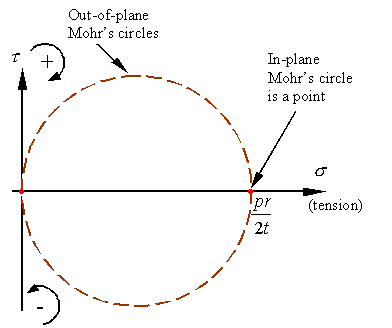As can be seen, the maximum and minimum normal stresses and maximum shear stress are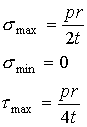As can also be seen, the maximum shear stress is on a 45o out-of-plane incline as shown in the figure.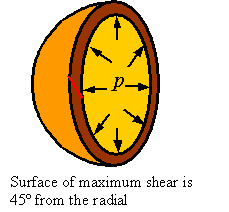Maximum stress on the inside surface:

The in and out-of-plane Mohr’s circles for a stress element taken from the inside surface of the pressure vessel will look as follows.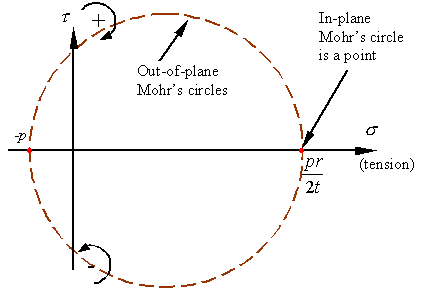As can be seen, the maximum and minimum normal stresses and maximum shear stress are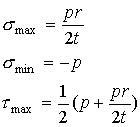The maximum shear stress is on a 45o out-of-plane inclined surface as shown in the figure.# Cylindrical pressure vessels:

Consider the stresses in a thin cylindrical pressure vessel of inner radius r and wall thickness t.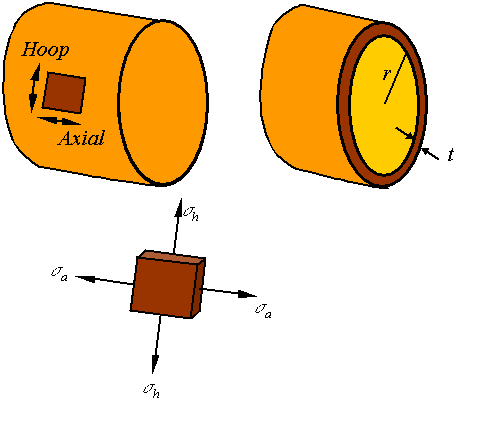Unlike the spherical pressure vessel for which the stress in all directions tangent to the sphere were the same, for a cylindrical pressure vessel the stressalong the axial direction is different from the stress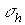along the hoop direction.

Axial stress:

To calculate the axial stress consider the stresses on a cross section of the cylinder as shown in the figure.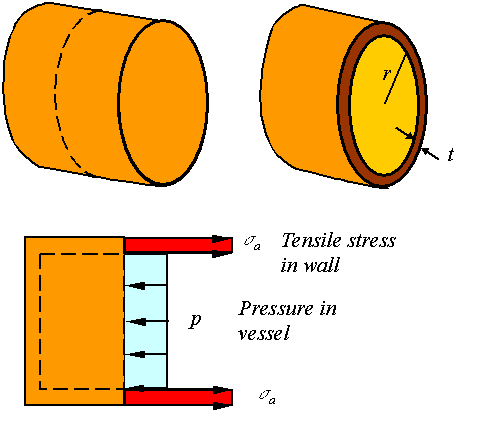Static equilibrium requires that the load generated from the tensile stress in the wall to be equal to the load applied by the pressure. Since the vessel is thin, the load due to the tensile stress in the wall is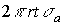. The load due to the pressure in the vessel is. Balancing these gives the expression for the stress in a spherical vessel as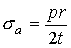Hoop stress:

To calculate the hoop stress consider the stresses on a section of the cylinder as shown in the figure.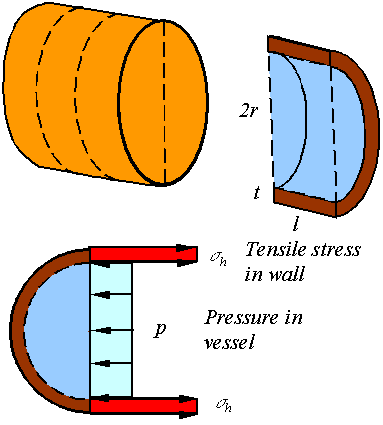Static equilibrium requires that the load generated from the tensile stress in the wall to be equal to the load applied by the pressure. The load due to the tensile stress in the wall is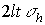. The load due to the pressure in the vessel is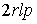. Balancing these gives the expression for the stress in a spherical vessel as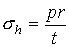Maximum stresses on the inside and outside surface:

The stress elements taken from the inside and outside surfaces of the cylindrical pressure vessel look as follows.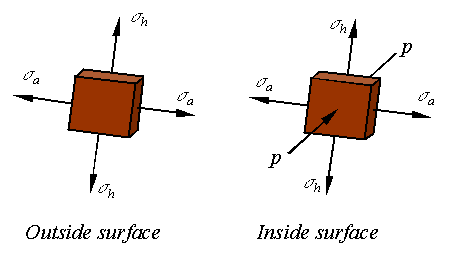The in and out-of-plane Mohr’s circles for a stress element taken from the outside surface of the pressure vessel will look as follows.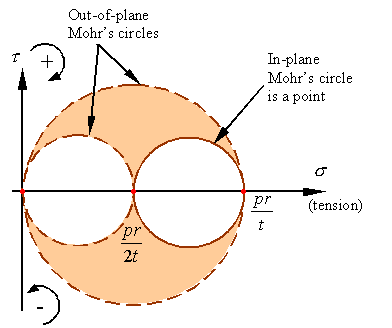As can be seen, the maximum and minimum normal stresses and maximum shear stress are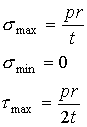The Mohr’s circles for an element from inside the pressure vessel will look as follows.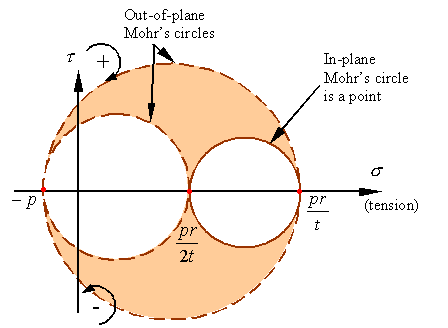As can be seen, the maximum and minimum normal stresses and maximum shear stress on the inner surface of the vessel is given by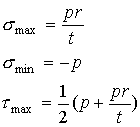The maximum shear stress is on the inner surface of the vessel and oriented on a surface that is 45o out of plane (similar to the spherical vessel).